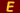# The Ecphorizer

#### Issue #07 (March 1982)

This contains a series of item previously appearing in the Intelligencer and extracted from either MacKenzie's Five Thousand Receipts published in 1851, or from a hand-written mathematics course book written by my great-grandfather in 1849. The following is from the math book:

"No. 16. I dropped a ball from Jackson's bridge
Its height above the stream to tell--
A pendulum two feet in length,
Made five vibrations while it fell:
Can you in numbers fairly show
How high this lofty bridge must be
Above the stream that glides below,
The feet and inches show to me?"

I know that the acceleration of gravity is 32 feet per second per second, and the distance fallen is half the acceleration multiplied by the time squared, so that in the first second the ball would drop 16 feet, at the end of the second second it would have fallen 64 feet, etc. (S = 1/2 AT). The answer was given as 244 feet, 10 inches, with the following:

"Note for the solution of the above problem: Divide the length of the given pendulum by the length of the pendulum vibrating seconds (39.2 inches) multiply the quotient by the square of the number of vibrations given in the question and that product by 16 (the number of feet that the ball falls in the first second of its descent) and the product will be the answer."

He took the length of the pendulum in inches for this solution. I do not know where the 39.2 inches came from, or why the square of the number of vibrations enters into it. Can any physicist/mathematician who reads this enlighten me?Mead, a retired financial executive, was for many years Treasurer of San Francisco Mensa. He travels frequently and collects antiques.